## Cutting speed (vc)

Check the item you want to calculate, input values in the two boxes, and then press the Calculate button.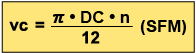vc (SFM) : Cutting Speed
DC (inch) : Drill Diameter
π (3.14) : Pi
n (min-1) : Rotational Speed of the Main Spindle

n(min-1)
DC(inch)
vc(SFM)

*Unit transformation (from "mm" to "m")

### ProblemWhat is the cutting speed when main axis spindle speed is 1350min-1 and drill diameter is .500inch ?

Substitute π=3.14, DC=.500inch, n=1350 into the formulae
vc=π×DC×n÷12=3.14×.500×1350÷12=176.6SFM
The cutting speed is 176.6SFM.

## Feed of the main spindle (vf)

Check the item you want to calculate, input values in the two boxes, and then press the Calculate button.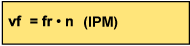vf (IPM)：Feed Speed of the Main Spindle (Z axis)
fr(IPR)：Feed per Revolution
n(min-1) ：Rotational Speed of the Main Spindle

fr(IPR)
n(min-1)
vf(IPM)

### Problem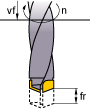What is the spindle feed (vf) when the feed per revolution is .008IPR and main axis spindle speed is 1350min-1 ?

Substitute fr=.008,n=1350 into the formulae
vf=fr×n=.008×1350=10.8IPM
The spindle feed is 10.8IPM.

## Drilling time (Tc)

Input the valuesTc (min) : Drilling Time
n (min-1) : Spindle Speed
ld (inch) : Hole Depth
fr (IPR): Feed per Revolution
i : Number of Holes

ld(inch)
i
n(min-1)
fr(IPR)
Tc(min)
sec

### Problem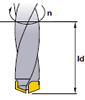What is the drilling time required for drilling a 1.2inch length hole in alloy steel at a cutting speed of 165SFM and a feed .006IPR ?

Spindle Speed n =(165×12)÷ (.59×3.14) = 1068.8min-1

Tc=(1.2×1)÷(1068.8×.006)=.187
=.187×60≒11.2sec.

## Cutting speed (vc)

Check the item you want to calculate, input values in the two boxes, and then press the Calculate button.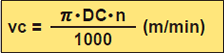*Divide by 1,000 to change to m from mm.

vc (m/min) : Cutting Speed
DC (mm) : Drill Diameter
π (3.14) : Pi
n (min-1) : Main Axis Spindle Speed

n(min-1)
DC(mm)
vc(m/min)

### ExampleWhat is the cutting speed when main axis spindle speed is 1350min-1 and drill diameter is 12mm ?

Substitute π=3.14, DC=12, n=1350 into the formula
vc=π×DC×n÷1000=3.14×12×1350÷1000=50.9m/min
The cutting speed is 50.9m/min.

## Feed of the main spindle (vf)

Check the item you want to calculate, input values in the two boxes, and then press the Calculate button.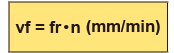vf(mm/min)：Feed Speed of the Main Spindle (Z axis)
fr(mm/rev)：Feed per Revolution
n(min-1) ：Main Axis Spindle Speed

fr(mm/rev)
n(min-1)
vf(mm/min)

### ExampleWhat is the spindle feed (vf) when the feed per revolution is 0.2mm/rev and main axis spindle speed is 1350min-1 ?

Substitute fr=0.2,n=1350 into the formula
vf=fr×n=0.2×1350=270mm/min
The spindle feed is 270mm/min.

## Drilling time (Tc)

Input the valuesTc (min) : Drilling Time
n (min-1) : Spindle Speed
ld (mm) : Hole Depth
fr (mm/rev): Feed per Revolution
i : Number of Holes

ld(mm)
i
n(min-1)
fr(mm/rev)
Tc(min)
second(s)

### ExampleWhat is the drilling time required for drilling a 30mm length hole in alloy steel (JIS SCM440) at a cutting speed of 50m/min and a feed 0.15mm/rev ?